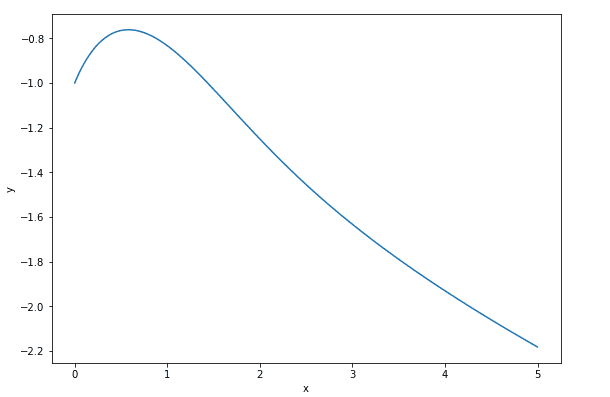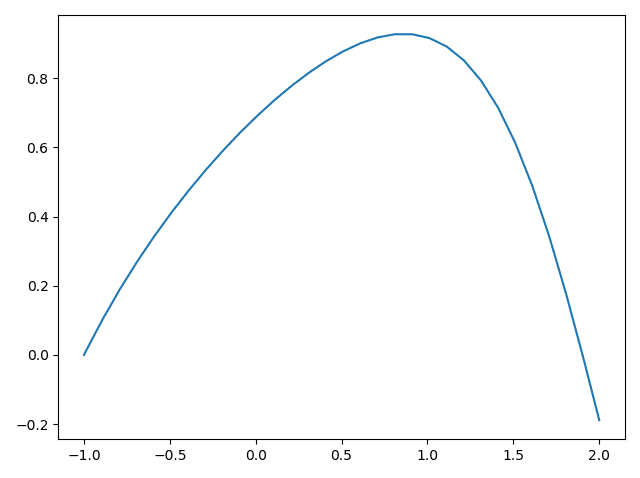# Euler Approximation Failure

• JessicaHelena

#### JessicaHelena

Homework Statement
For the DE ##\frac{dy}{dx} = y^2-x,## the long term behaviour of the Euler approximations with step sizes ##h = 0.5, 0.25, 0.125## with initial condition ##(-1,0)## all tend to ##\infty##. However, the long term behaviour of the actual solution with the same initial condition actually tends to ##-\infty##.

What would I call this failure——failure of self-consistency, failure of convergence, failure of structural stability, or failure of stability?
Relevant Equations
N/A
I had thought it would be failure of structural stability since in structural stability qualitative behavior of the trajectories is unaffected by small perturbations, and here, even tiny deviations using ##h## values resulted in huge effects. However, apparently that's not the case, and I'm not sure which failure this falls under...

I cannot see a solution which tends to ##-\infty ##
https://www.wolframalpha.com/input/?i=y'=y^2-x+
Not with ## y(0) = 1 ##, but with ## y(0) = -1 ## it does...Python:
import numpy as np
import matplotlib.pyplot as plt
from scipy.integrate import odeint

# ODE
def odeFunc(y, x):
return y * y - x

# Initial conditions
y_0 = -1.0

start = 0.0
stop = 5.0

step = 0.001

listOfXValues = np.arange(start, stop, step)

arrayOfYValues = odeint(odeFunc, y_0, listOfXValues)

# Plot results
fig = plt.figure(1, figsize=(8, 16))

ax1.plot(listOfXValues, arrayOfYValues[:,0])
ax1.set_xlabel('x')
ax1.set_ylabel('y')

plt.tight_layout()
plt.show()

You have correctly described the phenomenon i.e. a small perturbation of the initial conditions resulting in a large difference in the trajectory of the solution, but this is not a description of structural instability. Revise the definitions and it should be clearer which one fits.

Last edited:
•WWGD
@fresh_42 and @pbuk
Thank you for your inputs! If I understood the materials fresh_42 provided me right, would it then just be a failure of stability? In fact, I don't think I see the words self-consistency, or convergence thrown around in those documents a whole lot, and googling didn't particularly help me either.

I am not sure we are heading in the right direction here, and IMHO that's because the question (as you have stated it) is misleading. The reason that you end up with the wrong trajectory as ## x \to \infty ## is nothing to do with any long-term instability of the DE, it is due to what goes wrong with the very first step!

To understand this, look at the expression for the error term in Euler's method (compare the Taylor series expansion with the first term that is not included in Euler's method) and calculate this at (0 + h, -1).

Edit: whilst radius of convergence is a good thing to revise, I'm not sure it helps you answer the question. But again, I don't think it's a very helpful question - if the learning point is supposed to be that Euler's method converges very slowly then there are much better ways of teaching that; I would integrate cos(x) in the region of (0, 0) where it is easy to plot the exact solution and show how the not particularly rapid reduction of the slope from 1 means that Euler's method is doomed to failure even over a fairly short interval.

Last edited:
•atyy and fresh_42
Could it be an example of what is called a "stiff" differential equation?

I think there may be some ambiguity in the use of the word "stability" in this discussion. In a stiff DE it's not the solution but the numerical algorithm which is unstable. Euler's method does not do well on such equations, and predictor-corrector methods do much better.

This problem would not usually be considered stiff but nearly all systems look stiff when you are using Euler's method! That is why I suggested calculating the error term
## y' = y^2 - x \implies y''(x) = 2yy' - 1 = 2y^3 - 2xy - 1 ## so at (0, -1) we have the error term ## O(\frac{-h^2}{2!}y''(0)) = O(\frac{3h^2}{2}) ##. So with a step size of 0.5 our first step takes us to (0, -0.5) with a y error of ≈0.375! ##
But why not choose a function with a simpler analytic solution and just consider the first couple of steps to show this point?

^I thought the initial condition was (-1,0)
it has a simple analytic solution
let u'+y u=0
to obtain the Airy equation or the Stokes equation
^^
stiff is subjective
this does not appear to be particularly stiff
Euler's methods is pretty unstable

^I thought the initial condition was (-1,0)
Oops, I must have misread it - doesn't change anything much though, the step size is still far too large (the first step with size 0.5 is now (-0.5, 0.5) with a y-error of ≈ 0.125).it has a simple analytic solution
let u'+y u=0
to obtain the Airy equation or the Stokes equation
^^
Not sure I follow this, are you saying make a substitution ## u = y' ##? I get $$u' = y'' = 2yy' - 1$$ $$u' - 2yu = -1$$ which is not quite an Airy equation.

I checked those flows at values for ##x \sim 20## on two different websites. It seems that the algorithm depends on implementation, too, i.e. differs the farther away from the initial value we get.

^^
u'+u y=0
u'=-u y
so
(u'+u y)'=0
u'+(u y)'=0
u''+u'y+u y'=0
but
u'=-u y
so
u''+(-u y)y+u y'=0
u''+u(-y^2+y')=0
u''+(-y^2+y')u=0
but
y'=y^2-x
so
u''+(-y^2+y^2-x)u=0
u''-x u==0
the Airy equation or the Stokes equation

•pbuk